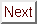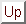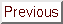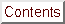Next: Multiband and Anisotropic Up: Possible Interpretations of Previous: Dimensional Crossover Models

## Critical Field Enhancement in Isotropic Superconductors

One often hears the statement that large spin-orbit scattering increases Hc2(T) in a high-field superconductor. Since the spin-orbit interaction increases rapidly as a function of the atomic number Z, and since Hg is a high-Z element, it is logical to ask whether spin-orbit scattering could enhance Hc2 in C4KHg. The answer is no, since it turns out that the spin-orbit ``enhancement'' only affects materials whose critical fields have already been depressed by Pauli limiting effects.[262,186] ``Pauli limiting'' refers to the upper bound on Hc2(T) from spin-susceptibility (Pauli paramagnetism) effects.[45,38] Superconductors close to their Pauli limit have critical fields which are depressed from the Maki-deGennes temperature dependence. Paramagnetic limiting also affects the angular dependence of the critical field.[9,53] High rates of spin-orbit scattering can increase the susceptibility of the superconducting state to that of the normal state, and thus eliminate Pauli-limiting effects. Thus spin-orbit scattering and Pauli limiting effectively cancel one another out, and the final result of both effects is the same Maki-deGennes curve already discussed in Section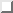. Since the two mechanisms cancel, and since spin-orbit scattering has no major impact for a superconductivity far from the paramagnetic limit, spin-orbit scattering cannot give a reduced field h*(0) > 0.7, and cannot explain the enhanced linearity of Hc2(T) observed in GIC's.

While on the subject of electron spin, it is worth mentioning that GIC's appear to be far from the paramagnetic limit, as would be expected for low-critical field materials. The reason is that orbital pair-breaking effects are strong enough in GIC superconductors that they are far from the spin-susceptibility ceiling on Hc2. The small values of the Maki alpha parameter for GIC's clearly demonstrate the validity of this statement, since spin contributions to the energy of a superconductor become important when alpha >= 1. WHH provided two ways of calculating alpha:Here Gamma is the linear specific heat coefficient, and rhon is the normal-state dc resistivity. In Eqn., m is the bare electron mass, not the effective mass, since it comes from ehbar/2 muBc. For C4KHg, the best method is to use rhoa to calculate alpha for vecH || ^c, and sqrtrhoa rhoc to calculate alpha for vecH _|_ ^c. The parameters used were rhoc = 0.2 milliohm-cm at 4.2 K, and Gamma = 0.95 millijoules / (mol K2). rhoa at 4.2 K for C4KHg (which has not been reported) was estimated by assuming a resistivity anisotropy of 280, the same as the measured resistivity anisotropy at 100 K, the lowest temperature at which published rhoa data exist. Values of alpha obtained from both halves of Eqn.are given in Table, which demonstrates that C4KHg is two to three orders of magnitude away from Pauli-limiting regime. With like assumptions, similar calculations for other GIC's give alpha's of the same order of magnitude. Therefore spin-orbit scattering has no impact on the critical fields of C4KHg.Table: Comparison for C4KHg of two different methods for determination of Maki's alpha parameter. The orientation indicated is that of the applied magnetic field. In parentheses it is noted which of the two halves of Eqn.was used.

WHH say about Eqn.that ``It is a test of the applicability of our model for the superconductor that these two determinations of alpha should agree.'' Considering the crudeness of the assumptions made in the estimation of rho, the agreement in Tablebetween the two determinations of alpha is quite good. This is an indication that even though superconductivity in GIC's has some anomalous aspects, it still is explained by the same basic electron-phonon coupling mechanism that applies to isotropic superconductors. Therefore, in our search for models to explain the enhanced critical fields of GIC's, models with truly exotic coupling schemes ( e.g., plasmons and excitons) can safely be ignored.

Another factor that contributes to critical field enhancement in isotropic superconductors besides spin-orbit scattering is strong-coupling effects. ``Strong-coupling'' refers to the case of a large electron-phonon interaction. The strength of electron-phonon coupling is measured by the size of the dimensionless parameter Lambdaep, the same electron-phonon parameter that appears in the dressed density-of-states found from specific-heat measurements. Note that Lambdaep is not related to the magnetic-field penetration depth Lambda (see Sectionfor a discussion of the penetration depth). McMillan calculated Tc in terms of Lambdaep and mu *, the Coulomb pseudopotential of Morel and Anderson. He found:where thetaD is the Debye temperature. Using a typical number of mu* = 0.1,, one can solve for Lambdaep:The results of this calculation for the superconducting GIC's whose Debye temperature has been measured are collected in Table. Lambdaep in GIC superconductors appears to be 0.4, about the same as in prototypical weak-coupling superconductors like aluminum and zinc. This is in keeping with one's expectations for a material with a rather low Tc of about 1-2 K.Table: Values of Lambdaep, the electron-phonon coupling parameter, for GIC superconductors. Tc = 0.73 K is used for C4KHg since no transition was observed down to 0.8 K during the specific-heat measurement. Values of Lambdaep for the KH-GIC's are gathered in Table.

In amorphous superconductors, strong-coupling effects can cause extended linearity at low temperatures. However, the values of Lambdaep quoted above eliminate the possibility that GIC superconductors are subject to any critical field field enhancement from strong-coupling effects, since these effects are important only for Lambdaep >= 1. Furthermore, since the strong-coupling enhancement is larger near Tc than at low t,[53,50] strong-coupling effects tend to actually decrease h*(0), the reduced field at zero temperature.

Recently some theories of ultra-strong coupling superconductivity have been published, inspired by the advent of high-temperature superconductivity.[31,162] Some of these models find positive curvature of Hc2(t). Bulaevskii and Dolgov find that h*(0) = 0.45sqrtLambdaep for Lambdaep >> 1. Marsiglio and Carbotte find h*(0) about 1.6, but only when Tc thetaD. These models are clearly not applicable to superconductivity in known GIC's.

For GIC's, a more relevant consideration than strong-coupling is inhomogeneity. From both structural[123,246] and superconducting[206,55] studies, there is abundant evidence for the coexistence of multiple phases in C4KHg. This evidence is discussed in detail in Chapter. As far as critical field experiments go, this multiphase behavior is important because of the possibility that inhomogeneity is the cause of the enhanced linearity of the critical fields of C4KHg. Carter and coauthors developed a model for the case of multiphase materials which contain both an equilibrium and higher free-energy phase. The model treats the inhomogeneity by allowing the superconductor to have a distribution of diffusivities described by a function P(D). Then, instead of the Maki-deGennes equation (Eqn.) for the critical fields of dirty superconductors, one uses:where all the symbols are the same as before, and psi is the digamma function. Carter et al. found that by widening the distribution P(D) from a Delta-function (implied by the choice of a single D value) to a broad hump that they could produce both positive curvature and h*(0) 0.85. By skewing the distribution P(D) to low D, they could even get h*(0) > 0.9. The results of their calculations are shown in Fig., where h* (called h in the axis label) is plotted versus t as a function of the normalized diffusivity distribution function, Q(y). Q(y) == D ave P(D), where Dave is the mean diffusivity, and y == D/ Dave.Figure: Extended critical field linearity due to small-scale sample inhomogeneity. From a calculation by Carter and colleagues. The plots are of reduced field versus reduced temperature for several different normalized diffusivity distribution functions Q(y). Q(y) == DaveP(D), where P(D) is the distribution function for diffusivity, Dave is the average diffusivity, and y == D/D ave. In the lower plot, a P(D) skewed to lower diffusivities produces an even greater critical field enhancement at low temperatures. The index n refers to the power of the linear factor used to skew the symmetric distribution.

The physical cause of the inhomogeneity-related enhancement has to do with the temperature dependence of xi, which is the approximate radius of a normal vortex in a superconductor. At T Tc, xi is large, so that vortices must extend over both high-D and low-D regions in the material. At low t, where xi has grown considerably smaller, the material can save some condensation energy by preferentially packing the vortices into the low-D regions with higher critical fields. As a result, when xi(T) becomes on the order of the domain size, Hc2 will turn upward.

Does this model offer an explanation of positive curvature and enhanced linearity in the critical fields of GIC's? Clearly the inhomogeneity interpretation has some appealing features for C4KHg, but it also has some problems. One is that among the GIC superconductors, multiphase behavior has been observed only in C4KHg. As is discussed in Chapter, C4KHg is remarkable among the superconducting GIC's for the wide range of Tc's it exhibits (from 0.7 to 1.6 K), and because it undergoes what is apparently an ordering transition under the influence of small perturbations (hydrogenation and small hydrostatic pressure). These features are not observed for other GIC's, which have well-defined transition temperatures and show no unusual behavior under pressure.[116,55] It does not seem to make sense to attribute the anomalies in C4KHg to a different cause than the deviations seen in other GIC's, especially considering that the other GIC's show larger anomalies (see Fig.).

Even if one were willing to assume separate causes for the enhanced critical fields of the various GIC's, it is not clear that the model of Carter and colleagues would be applicable. The problem is that their model makes the (reasonable) assumption that microscopically inhomogeneous superconductors will be in the dirty limit, where the Maki-deGennes equation is applicable. However, C4KHg appears to be fairly ``clean,'' at least for in-plane transport. The standard way to quantify cleanliness in a superconductor is to calculate the parameter Lambdatr == 0.882 xi0 / l. Here xi0 == 0.18 hbar vF/ kB Tc is the Pippard coherence length, and l is the mean-free path. For C4KHg, xi0 is roughly 9000 Å, and la, the in-plane mean-free path, is about 9100 Å. (These numbers were obtained from Shubnikov-deHaas data using standard rigid-band analysis, as demonstrated in Appendix.) Since la xi0, the dimensionless parameter Lambdatr == 0.88 xi0/l 0.86 for in-plane transport. Lambdatr < 1 is indicative of fairly clean behavior, so this is an indication that inhomogeneities are not likely to be the cause of enhanced linearity, at least for vecH || ^c. However, this calculation does not rule out a diffusivity-variation influence on vecH _|_ ^c. Transport is expected to be much dirtier along ^c, where the resistivity is about 300 times higher than in-plane. Lambdatr is estimated very roughly to be about 50 for c-axis transport by assuming a spherical band. (No well-bounded number is available since the Shubnikov-deHaas data give no information about the intercalant bands. c-axis transport in GIC's is discussed in Section.)

In summary, use of the inhomogeneity model for enhanced Hc2 can be justified for C4KHg for vecH _|_ ^c, but it is hard to justify for vecH || ^c, or for other superconducting GIC's. Therefore the most sensible conclusion is that, while the factors discussed by Carter et al. may play a role in C4KHg, they probably do not have a dominant effect on Hc2(T).

Finally, to round out the discussion of critical field enhancement in isotropic superconductors, mention should be made of the anomalous Hc2(T) behavior found in heavy-fermion superconductors. These unusual materials exhibit both positive curvature and large values of h*(0). Any explanation of these phenomena must remain tentative since the basic physics of these compounds is still controversial, but recently an interesting model has been proposed by DeLong et al. The explanation is based on the observation that dHc2/dT propto rho in the usual WHH model, as shown in Eqn.. If the normal-state resistance rho is assumed independent of field up to Hc2, then one gets the usual WHH result h*(0) 0.7. However, if there is a very strong magnetoresistance (the manifestation of a field-dependent pairing interaction), then the slope formula shows that h*(0) can exceed 0.7 by a factor of Delta rho(Hc2) / rho. Here Delta rho(Hc2) / rho is the magnetoresistance at the field Hc2; the normal state resistivity at zero-field is extrapolated from above Tc. This formalism may have wide applicability to superconductors with large or anomalous magnetoresistance; however, it appears unlikely to help much in the case of GIC's, since the critical fields are small enough that the magnitude of Delta rho(Hc2)/rho is anticipated to be 0. The magnetoresistance of the KHg-GIC's was observed by Timp and coworkers, who reported nothing extraordinary.

Four causes of critical field enhancement in isotropic superconductors have been discussed: spin-orbit scattering, strong-coupling effects, inhomogeneities, and magnetoresistance. Of these, only the multiphase-superconductor model of Carter et al. is thought to be relevant to GIC superconductors. Inhomogeneity effects could play a role for vecH _|_ ^c, but except for C4KHg, there is no hard evidence for multiphase behavior in other superconducting GIC's except for C4KHx.[79,232]

The extremely anisotropic nature of superconductivity in GIC's (1/epsilon as high as 47) provides motivation to consider models of positive curvature and enhanced linearity which treat orientation-dependent effects as central. Some of these models are considered in the next section.Next: Multiband and Anisotropic Up: Possible Interpretations of Previous: Dimensional Crossover Models

alchaiken@gmail.com (Alison Chaiken)
Wed Oct 11 22:59:57 PDT 1995